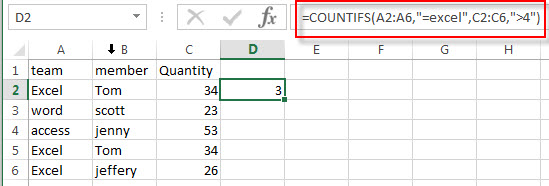# Excel COUNTIFS function

This post will teach you how to use Excel COUNTIFS function with syntax and examples in Microsoft excel.

Description

The Excel COUNTIFS function returns the count of cells in a range that meet one or more criteria. It allows to use the criteria with the numeric value or text string, which may be contain the wildcards in text-related criteria, and also support to use the logical operator to build an expression statement for criteria. You also should know that the COUNTIFS function is not case-sensitive.

The COUNTIFS function is a build-in function in Microsoft Excel and it is categorized as a Statistical Function.

The COUNTIFS function is available in Excel 2016, excel 2013, excel 2010, excel 2007, excel 2003, excel XP, excel 2000, excel 2011 for Mac.

Syntax

The syntax of the COUNTIFS function is as below:

`= COUNTIFS(criteria_range1, criteria1, [criteria_range2, criteria2]…)`

Where the COUNTIFS function arguments are:

• criteria_range1 –This is a required argument.  the first range in which to apply the associated criteria.
• criteria1 – This is a required argument.  the first critiria to use on criteria_range1
• criteria_range2, criteria2, …    Optional. Additional ranges and their associated criteria. Up to 127 range/criteria pairs are allowed.

Note:

• Each range’s criteria is applied one cell at a time. If all of the first cells meet their associated criteria, the count increases by 1. If all of the second cells meet their associated criteria, the count increases by 1 again, and so on until all of the cells are evaluated.
• If the criteria argument is a reference to an empty cell, the COUNTIFS function treats the empty cell as a 0 value.
• You can use the wildcard characters— the question mark (?) and asterisk (*) — in criteria. A question mark matches any single character, and an asterisk matches any sequence of characters. If you want to find an actual question mark or asterisk, type a tilde (~) before the character.

Excel COUNTIFS Function Examples

The below examples will show you how to use Excel COUNTIFS Function to count cells that match multiple criteria.

#1 To count how many rows have numbers that are greater than 50 in range C2:C6, and also the product name is Excel in range A2:A6 , just using the following excel formula:

`=COUNTIFS(A2:A6,"=excel",C2:C6,">4")`### More Excel Countifs Function Examples

• COUNTIF with Multiple Criteria
To count the number based on multiple criteria in the same range or column in Excel, you can create a formula based on the SUMPRODUCT function and the COUNTIF function.….